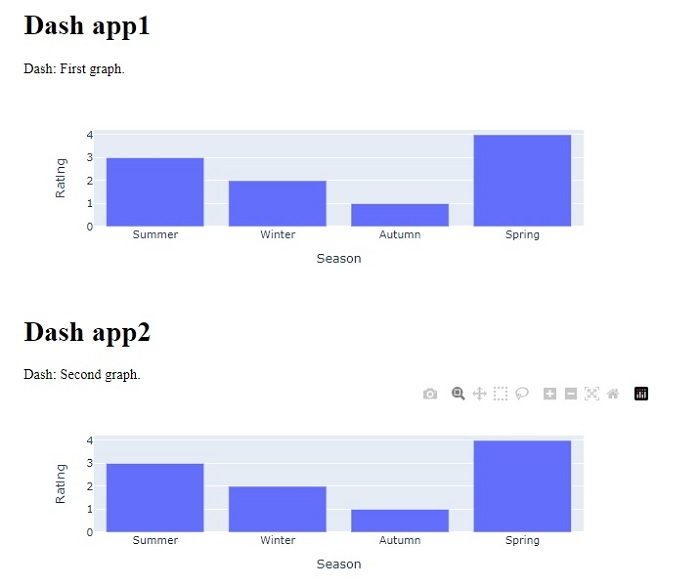# How to add multiple graphs to a Plotly Dash app on a single browser page in Python Plotly?

Plotly is an open-source plotting library in Python that can generate several different types of charts. Python users can use Plotly to create interactive web-based visualizations that can be displayed in Jupyter notebooks, saved to standalone HTML files, or served as a part of web applications using Dash. Plotly can also be used in static document publishing and desktop editors such as PyCharm and Spyder.

Dash is a Python framework and it is used to create interactive web-based dashboard applications. The dash library adds all the required libraries to web based dashboard applications.

In this tutorial, we will show how you can add multiple graphs to a Plotly Dash app on a single browser page. Follow the steps given below to generate multiple Dash apps on a single page.

Step 1

Import the Dash library.

import dash


Step 2

Import the Dash core components, dcc and html.

from dash import dcc,html


Step 3

Import the following Dash dependencies.

from dash.dependencies import Input, Output


Step 4

Import the plotly.express module and alias as px.

import plotly.express as px


Step 5

Generate a dataset using the Pandas module

import pandas as pd
df_bar = pd.DataFrame({
"Season": ["Summer", "Winter", "Autumn", "Spring"],
"Rating": [3,2,1,4]
})


Step 6

Generate a bar chart using the following values and store it inside the "fig" variable.

fig = px.bar(df_bar, x="Season", y="Rating", barmode="group")


Step 7

Create the main function to run the App server using the following command −

app = dash.Dash(__name__)
if __name__ == '__main__':
app.run_server(debug=True)


Step 8

Generate the App layout for two different HTML children in two "div" sections.

app.layout = html.Div(children=[
# elements from the top of the page
html.Div([html.H1(children='Dash app1'),
html.Div(children='''Dash: First graph.'''),
dcc.Graph(id='graph1',figure=fig),]),

# New Div for all elements in the new 'row' of the page
html.Div([
html.H1(children='Dash app2'),
html.Div(children='''Dash: Second graph.'''),
dcc.Graph(id='graph2',figure=fig),
]),
])


## Example

Here is the complete code to create multiple graphs on a single web page in Dash −

import dash
from dash import dcc,html
from dash.dependencies import Input, Output

import pandas as pd
import plotly.express as px
app = dash.Dash(__name__)
df_bar = pd.DataFrame({
"Season": ["Summer", "Winter", "Autumn", "Spring"],
"Rating": [3,2,1,4]
})

fig = px.bar(df_bar, x="Season", y="Rating", barmode="group")
app.layout = html.Div(children=[
# elements from the top of the page
html.Div([
html.H1(children='Dash app1'),
html.Div(children='''
Dash: First graph.'''),

dcc.Graph(
id='graph1',
figure=fig
),
]),
# New Div for all elements in the new 'row' of the page
html.Div([
html.H1(children='Dash app2'),
html.Div(children='''
Dash: Second graph. '''),

dcc.Graph(
id='graph2',
figure=fig
),
]),
])

if __name__ == '__main__':
app.run_server(debug=True)


## Output

When you execute the above program, you will get the following output on the console −

Dash is running on http://127.0.0.1:8050/
* Debug mode: on


Upon clicking the URL, you will be redirected to the browser which will show the following output −Updated on: 21-Oct-2022

4K+ Views